## RD Sharma Class 9 Solutions Chapter 2 Exponents of Real Numbers VSAQS

These Solutions are part of RD Sharma Class 9 Solutions. Here we have given RD Sharma Class 9 Solutions Chapter 2 Exponents of Real Numbers VSAQS

Question 1.
Write (625)1/4 in decimal form.
Solution: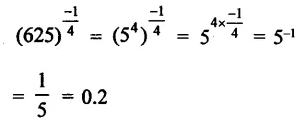Question 2.
State the product law of exponents:
Solution:
xm x xn = xm +n

Question 3.
State the quotient law of exponents.
Solution:
xm ÷ xn = xm -n

Question 4.
State the power law of exponents.
Solution:
(xm)n =xm x n = xmn

Question 5.
If 24 x 42 – 16x, then find the value of x.
Solution:Question 6.Solution: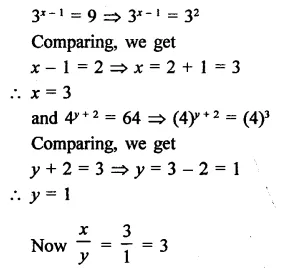Question 7.
Write the value of $$\sqrt [ 3 ]{ 7 }$$  x $$\sqrt [ 3 ]{ 49 }$$ .
Solution: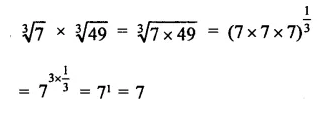Question 8.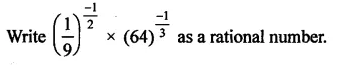Solution: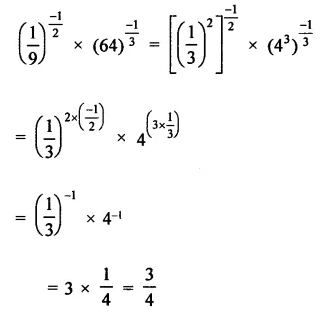Question 9.
Write the value of $$\sqrt [ 3 ]{ 125×27 }$$
Solution: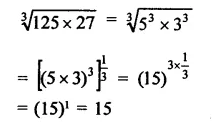Question 10.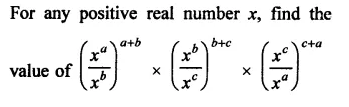Solution: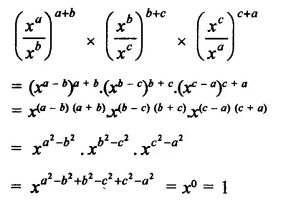Question 11.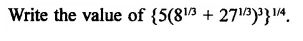Solution: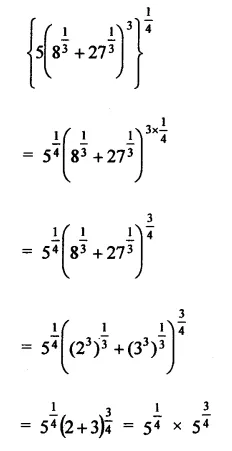Question 12.Solution: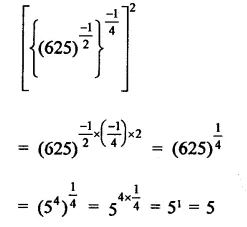Question 13.Solution:Question 14.
If (x – 1)3 = 8, what is the value of (x + 1)2?
Solution:Hope given RD Sharma Class 9 Solutions Chapter 2 Exponents of Real Numbers VSAQS are helpful to complete your math homework.

If you have any doubts, please comment below. Learn Insta try to provide online math tutoring for you.